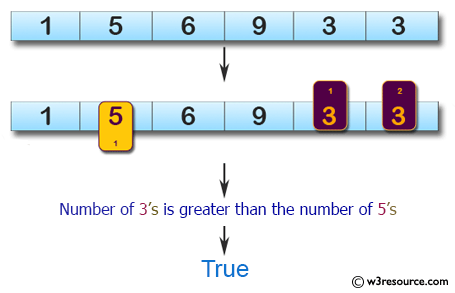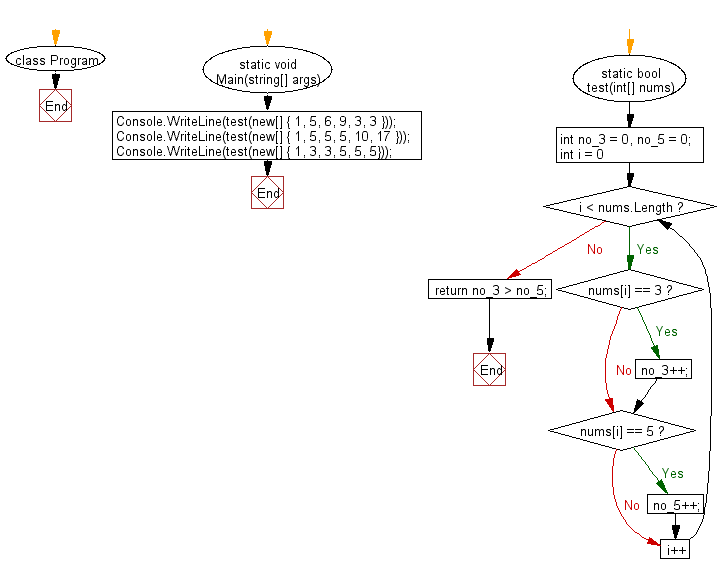﻿ C# - Number of 3 greater than the number of 5 in a array# C# Sharp Basic Algorithm Exercises: Check whether the number of 3's is greater than the number of 5's

## C# Sharp Basic Algorithm: Exercise-116 with Solution

Write a C# Sharp program to check whether the number of 3's is greater than the number of 5's.

Pictorial Presentation:Sample Solution:

C# Sharp Code:

``````using System;
namespace exercises
{
class Program
{
static void Main(string[] args)
{
Console.WriteLine(test(new[] { 1, 5, 6, 9, 3, 3 }));
Console.WriteLine(test(new[] { 1, 5, 5, 5, 10, 17 }));
Console.WriteLine(test(new[] { 1, 3, 3, 5, 5, 5}));
}
static bool test(int[] nums)
{
int no_3 = 0, no_5 = 0;

for (int i = 0; i < nums.Length; i++)
{
if (nums[i] == 3) no_3++;
if (nums[i] == 5) no_5++;
}

return no_3 > no_5;
}
}
}
```
```

Sample Output:

```True
False
False```

Flowchart:C# Sharp Code Editor:

Improve this sample solution and post your code through Disqus

What is the difficulty level of this exercise?

Test your Programming skills with w3resource's quiz.

﻿